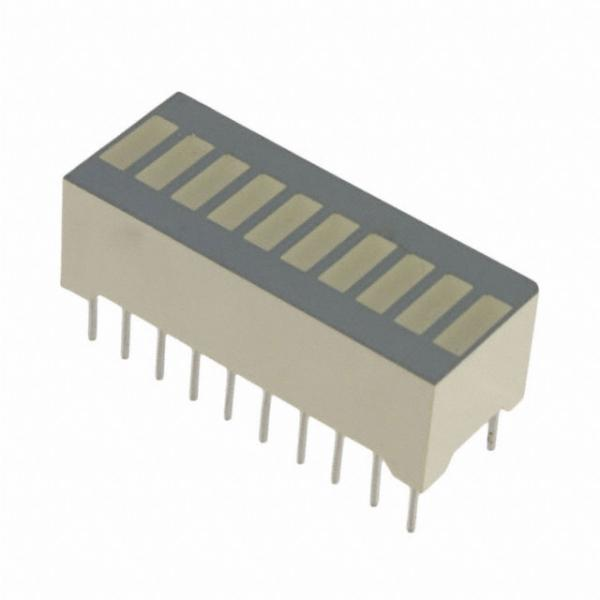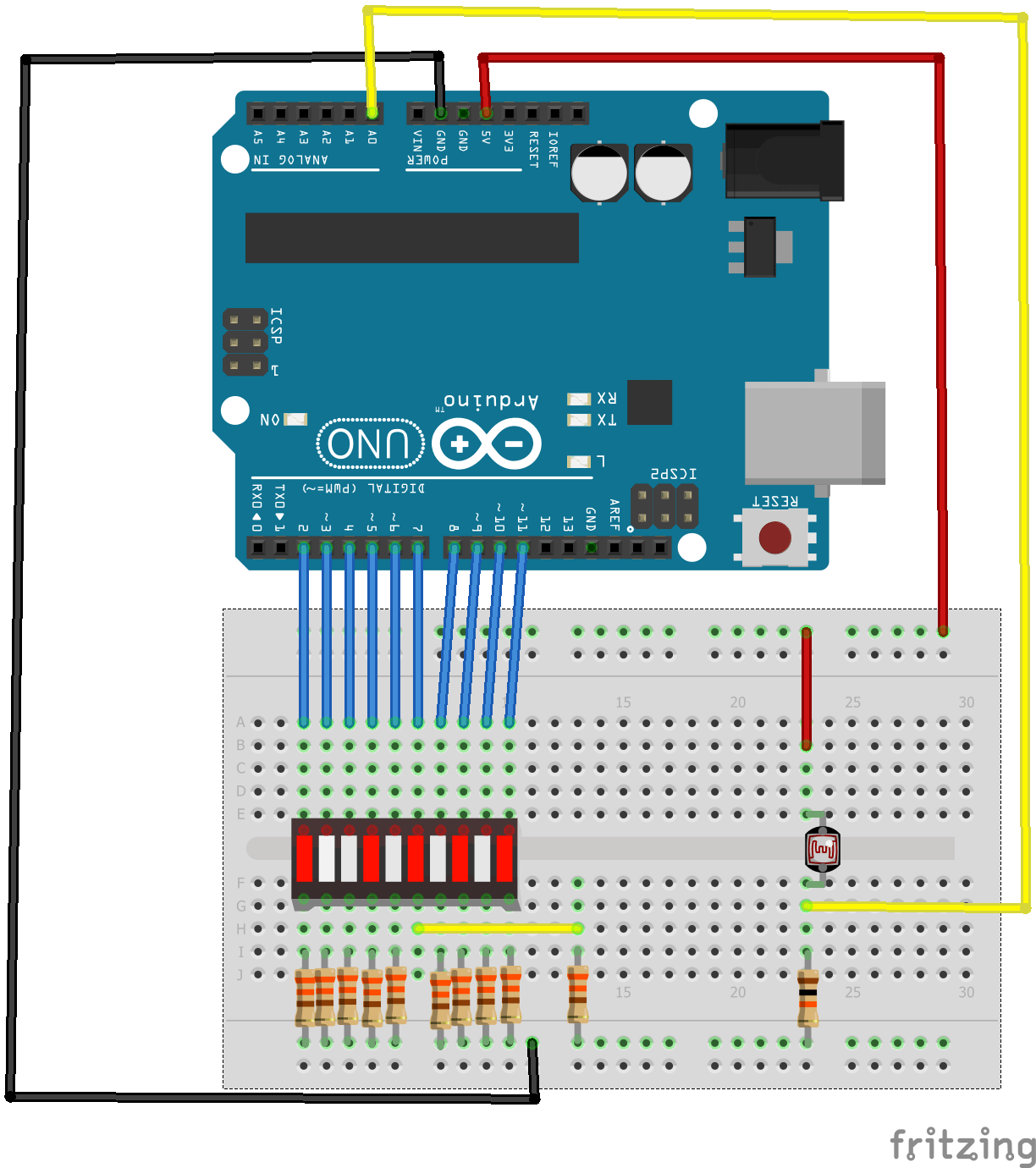## Arduino simple light meter

In this example we will connect and LDR up to our Arduino and display the reading on a bargraph display, think of this as a low cost type of light meter.

A typical bargraph display looks like the one below, mine was labelled as a FJB10RParts List

You will need the following and also breadboard and cables.

AmountPart TypeProperties
1LED_BARGRAPH_10segments 10; package led_bargaph_10
1Arduino Uno (Rev3)type Arduino UNO (Rev3)
10330Ω Resistorresistance 330Ω; tolerance ±5%; bands 4; package THT; pin spacing 400 mil
1Photocell (LDR)resistance@ luminance 16 kOhms@ 10 lux; package THT; resistance@ dark 300 kOhms@ 10 seconds
110kΩ Resistorresistance 10kΩ; tolerance ±5%; bands 4; package THT; pin spacing 400 mil

Layout

Here is the layout, I connected the output from the LDR circuit to A0 and used D2 to D11 to connect to the bargraph, obviously this uses a lot of I/O so that is something you may want to think aboutCode

Very basic example here

 Source code```void setup ()
{
for (int i = 2; i <=11; i ++)
{
pinMode (i, OUTPUT);
}
}

void loop ()
{
int k = map (ldrvalue, 0, 1000, 2, 11);
for (int j = 3; j <14; j ++)
{
if (j <= k)
digitalWrite (j, HIGH);
else
digitalWrite (j, LOW);
}
}```

Cover the LDR and shine light on it, you should varying amounts of LEDs on the bargraph lit

## Arduino PWM example

In this example we connect an LDR to analog 0 and depending on the value read in we then vary the brightness of an LED connected to Pin 9 using PWM.

The input read from the analog pins will be in the range 0 to 1023. But the PWM function has a the width parameter ranging from 0 to 25 so in this case we use the map() function to convert the values ranging from 0-1023 to 0-255.

Schematic

Code

```const int analogInPin = A0;  // Analog input pin that the potentiometer is attached to
const int PWMOutPin = 9; // Analog output pin that the LED is attached to
int sensorValue = 0;        // value read from the pot
int outputValue = 0;        // value output to the PWM (analog out)
void setup()
{
}
void loop()
{
// map it to the range of the analog out:
outputValue = map(sensorValue, 0, 1023, 0, 255);
// change the analog out value:
analogWrite(PWMOutPin, outputValue);
// wait 2 milliseconds for the a/d converter to settle
delay(2);
}
```

## Arduino and LDR example

In this example we connect a photoresistor to an Arduino, the value read from the photoresistor corresponds to the amount of light present. The photoresistor is connected to analog pin 0 in this example.

photoresistor (or light-dependent resistorLDR, or photo-conductive cell) is a light-controlled variable resistor. The resistance of a photoresistor decreases with increasing incident light intensity; in other words, it exhibits photoconductivity. A photoresistor can be applied in light-sensitive detector circuits, and light-activated and dark-activated switching circuits.

A photoresistor is made of a high resistance semiconductor. In the dark, a photoresistor can have a resistance as high as several megohms (MΩ), while in the light, a photoresistor can have a resistance as low as a few hundred ohms. If incident light on a photoresistor exceeds a certain frequency, photons absorbed by the semiconductor give bound electrons enough energy to jump into the conduction band. The resulting free electrons (and their hole partners) conduct electricity, thereby lowering resistance. The resistance range and sensitivity of a photoresistor can substantially differ among dissimilar devices. Moreover, unique photoresistors may react substantially differently to photons within certain wavelength bands.

A photoelectric device can be either intrinsic or extrinsic. An intrinsic semiconductor has its own charge carriers and is not an efficient semiconductor, for example, silicon. In intrinsic devices the only available electrons are in the valence band, and hence the photon must have enough energy to excite the electron across the entire bandgap. Extrinsic devices have impurities, also called dopants, added whose ground state energy is closer to the conduction band; since the electrons do not have as far to jump, lower energy photons (that is, longer wavelengths and lower frequencies) are sufficient to trigger the device. If a sample of silicon has some of its atoms replaced by phosphorus atoms (impurities), there will be extra electrons available for conduction. This is an example of an extrinsic semiconductor

A practical example could be a dark room sensor for photography, if the reading approached a critical level an alarm could be activated or even a night light

Here is our sensor, it was part of a 37 in 1 sensor kit, links at the bottom

Schematic

Code

In this example we simply output the reading via the serial port. You can monitor this in the Serial Port Monitor

 Source code```int sensorValue;

void setup()
{
Serial.begin(9600); // starts the serial port at 9600
}

void loop()
{
Serial.print(sensorValue, DEC); // prints the value read
Serial.print(” \n”); // prints a space between the numbers
delay(1000); // wait 100ms for next reading
}```

In this example we check the value returned by the photoresistor, if it drops beneath a certain value we switch the onboard LED on, if it goes above this value the LED will switch off

 Source code```int sensorValue;
int led = 13;

void setup()
{
pinMode(led, OUTPUT);
}

void loop()
{
//daylight was 700 – so lets detect a reading under 300
if (sensorValue < 300)
{
digitalWrite(led, HIGH);
}
else
{
digitalWrite(led, LOW);
}
delay(1000); // wait 100ms for next reading
}```